# 7.7: Stability of Alkenes

$$\newcommand{\vecs}{\overset { \rightharpoonup} {\mathbf{#1}} }$$ $$\newcommand{\vecd}{\overset{-\!-\!\rightharpoonup}{\vphantom{a}\smash {#1}}}$$$$\newcommand{\id}{\mathrm{id}}$$ $$\newcommand{\Span}{\mathrm{span}}$$ $$\newcommand{\kernel}{\mathrm{null}\,}$$ $$\newcommand{\range}{\mathrm{range}\,}$$ $$\newcommand{\RealPart}{\mathrm{Re}}$$ $$\newcommand{\ImaginaryPart}{\mathrm{Im}}$$ $$\newcommand{\Argument}{\mathrm{Arg}}$$ $$\newcommand{\norm}{\| #1 \|}$$ $$\newcommand{\inner}{\langle #1, #2 \rangle}$$ $$\newcommand{\Span}{\mathrm{span}}$$ $$\newcommand{\id}{\mathrm{id}}$$ $$\newcommand{\Span}{\mathrm{span}}$$ $$\newcommand{\kernel}{\mathrm{null}\,}$$ $$\newcommand{\range}{\mathrm{range}\,}$$ $$\newcommand{\RealPart}{\mathrm{Re}}$$ $$\newcommand{\ImaginaryPart}{\mathrm{Im}}$$ $$\newcommand{\Argument}{\mathrm{Arg}}$$ $$\newcommand{\norm}{\| #1 \|}$$ $$\newcommand{\inner}{\langle #1, #2 \rangle}$$ $$\newcommand{\Span}{\mathrm{span}}$$$$\newcommand{\AA}{\unicode[.8,0]{x212B}}$$

##### Objectives

After completing this section, you should be able to

1. explain why cis alkenes are generally less stable than their trans isomers.
2. explain that catalytic reduction of a cis alkene produces the same alkane as the catalytic reduction of the trans isomer.
3. explain how heats of hydrogenation (ΔH°hydrog) can be used to show that cis alkenes are less stable than their trans isomers, and discuss, briefly, the limitations of this approach.
4. arrange a series of alkenes in order of increasing or decreasing stability.
5. describe, briefly, two of the hypotheses proposed to explain why alkene stability increases with increased substitution. [Note: This problem is a typical example of those instances in science where there is probably no single “correct” explanation for an observed phenomenon.]
##### Key Terms

Make certain that you can define, and use in context, the key terms below.

• catalytic hydrogenation
• heat of hydrogenation, (ΔH°hydrog)
• hyperconjugation
##### Study Notes

The two alkenes, cis-CH3CH=CHCH3 and (CH3)2C=CH2 have similar heats of hydrogenation (−120 kJ/mol and −119 kJ/mol, respectively), and are therefore of similar stability. However, they are both less stable than trans-CH3CH=CHCH3 (−116 kJ/mol).

You may wonder why an sp2 -sp3 bond is stronger than an sp3-sp3 bond. Bond strength depends on the efficiency with which orbitals can overlap. In general, s orbitals overlap more efficiently than do p orbitals; therefore, the s-s bond in the hydrogen molecule is stronger than the p-p bond in fluorine. In hybrid orbitals, the greater the s character of the orbital, the more efficiently it can overlap: an sp2 orbital, which has a 33% s character, can overlap more effectively than an sp3 orbital, with only 25% s character.

## Hydrogenation

Alkene hydrogenation is the addition of hydrogen gas (H2) to an alkene which saturates the bond and forms an alkane. Alkene hydrogenation reactions require a transition metal catalyst, such as Pt or Pd, to speed up the reaction. The hydrogenation reaction is used in this section to investigate the stability of alkenes, however, it will be discussed in greater detail in Section 8.7. Hydrogenation reactions are exothermic and the enthalpy change in this reaction is called the heat of hydrogenation (ΔH°hydrog). Since the double bond is breaking in this reaction, the energy released during hydrogenation is proportional to the energy in the double bond of the molecule. By comparing the heat of hydrogenations from a series of alkenes that produce the same alkane, a quantitative measure of relative alkene stabilities can be produced. These experiments will lead to an general understanding of structural features which tend to stabilize or destabilize alkenes.

## The Catalyst

A catalyst increases the reaction rate by lowering the activation energy of the reaction. Although the catalyst is not consumed in the reaction, it is required to accelerate the reaction sufficiently to be observed in a reasonable amount of time. Catalysts commonly used in alkene hydrogenation are: platinum, palladium, and nickel. The metal catalyst acts as a surface on which the reaction takes place. This increases the rate by putting the reactants in close proximity to each other, facilitating interactions between them. With this catalyst present, the sigma bond of H2 breaks, and the two hydrogen atoms instead bind to the metal (see #2 in the figure below). The $$\pi$$ bond of the alkene weakens as it also interacts with the metal (see #3 below).

Since both the reactants are bound to the metal catalyst, the hydrogen atoms can easily add, one at a time, to the previously double-bonded carbons (see #4 and #5 below). The position of both of the reactants bound to the catalyst makes it so the hydrogen atoms are only exposed to one side of the alkene. This explains why the hydrogen atoms add to same side of the molecule, called syn-addition.Figure $$\PageIndex{1}$$: Hydrogenation takes place in the presence of a metal catalyst.
##### Note

The catalyst remains intact and unchanged throughout the reaction.

## Heats of Hydrogenation

The stability of alkene can be determined by measuring the amount of energy associated with the hydrogenation of the molecule. Since the double bond is breaking in this reaction, the energy released in hydrogenation is proportional to the energy in the double bond of the molecule. This is a useful tool because heats of hydrogenation can be measured very accurately. The $$\Delta H^o$$ is usually around -30 kcal/mol for alkenes. Stability is simply a measure of energy. Lower energy molecules are more stable than higher energy molecules. More substituted alkenes are more stable than less substituted ones due to hyperconjugation. They have a lower heat of hydrogenation. The following illustrates stability of alkenes with various substituents:## Cis/Trans Isomers

Between cis and trans isomers of an alkene, the cis isomer tends to be less stable due to the molecular crowding created nonbonding interaction between two alky groups on the same side of the double bond. The crowding creates steric strain which distorts bond angles creating less effective bond orbital overlap and desabilizing the molecule. Steric strain has previously been seen in gauche interactions in Newman projections (Section 3.7) and 1,3-diaxial interactions in substituted cyclohexanes (Section 4.7). Steric strain is directly related to the size of the species being crowded. The difference in energy between cis and trans 2-butene is 5 kJ/mol, however, this difference would be greater if larger group were being held in the cis position. Two cis-tert-butyl group can create over 40 kJ/mol of steric strain.

See the following isomers of butene:Figure $$\PageIndex{2}$$: Trans-2-butene is the most stable because it has the lowest heat of hydrogenation.

## Alkene Stabilization by Alkyl Substituents

In general, the stability of an alkene increases with the number of alkyl substituents. This effect is due by the combination of two factors:

### Hyperconjugation

In classical valence-bond theory, electron delocalization can only occur by the parallel overlap of adjacent p orbitals. According to hyperconjugation theory, electron delocalization could also occur by the parallel overlap of p orbitals with adjacent hybridized orbitals participating in sigma bonds. This electron delocalization serves to stabilize the alkene. As the number of alkyl substituents increases, the number of sigma bonds available for hyperconjugation increases, and the alkene tends to become more stabilized. In the example of propene shown below, a p orbital from a sp2 hybridized carbon involved in the double bond interacts with a sp3 hybridized orbital participating in an adjacent C-H sigma bond.In a molecular orbital description of hyperconjugation, the electrons in sigma molecular orbitals (C-H or C-C) of alkyl substituens, interact with adjacent unpopulated non-bonding or antibonding molecular orbitals from the double bond. The interaction creates a bonding molecular orbital which extends over the four atom chain (C=C-C-H) involved in hyperconjugation. The expanded molecular orbital helps to stabilize the double bond.### Bond Stability

Bond strengths play an important part in determining the overall stability of a molecule. A C-C bond between a sp3 carbon and a sp2 carbon is slightly stronger than a C-C bond between two sp3 carbons. Increasing the number alkyl substituents of a double bond also increases the number of sp3-sp2 C-C bonds making the alkene more stable. This is idea can be clearly seen when comparing the isomers 1-butene and 2-butene. The molecule 1-butene is monosubstituted and contains a sp3-sp3 C-C and a sp3-sp2 C-C bond. The disubstituted, 2-butene, contains 2 sp3-sp2 C-C bonds which contributes to its greater stability.##### Note

In cycloalkenes smaller than cyclooctene, the cis isomers are more stable than the trans as a result of ring strain.

##### Exercise $$\PageIndex{1}$$

1) Of the three following isomers which would be expected to be the most stable?

a)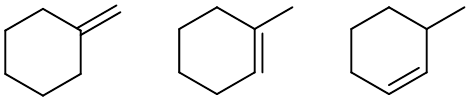b)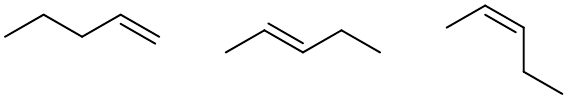c)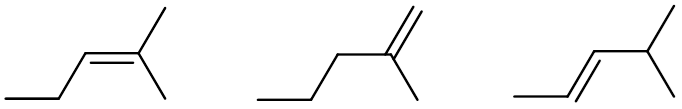1)

a)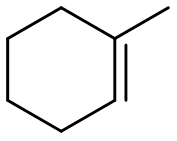b)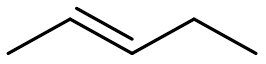c)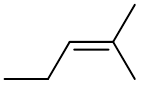##### Exercise $$\PageIndex{2}$$

3-Bromobut-1-ene reacts with hydrogen gas in the presence of a platinum catalyst. What is the name of the product?2-Bromobutane (numbering changes when alkene is no longer present)##### Exercise $$\PageIndex{3}$$

Cyclohexene reacts with hydrogen gas in the presence of a palladium catalyst. What is the name of the product?Cyclohexane##### Exercise $$\PageIndex{4}$$

What is the stereochemistry (syn or anti addition) of an alkene hydrogenation reaction?

##### Exercise $$\PageIndex{5}$$

When looking at their heats of hydrogenation, is the cis or the trans isomer generally more stable?

Trans

##### Exercise $$\PageIndex{1}$$

Show the product for the following